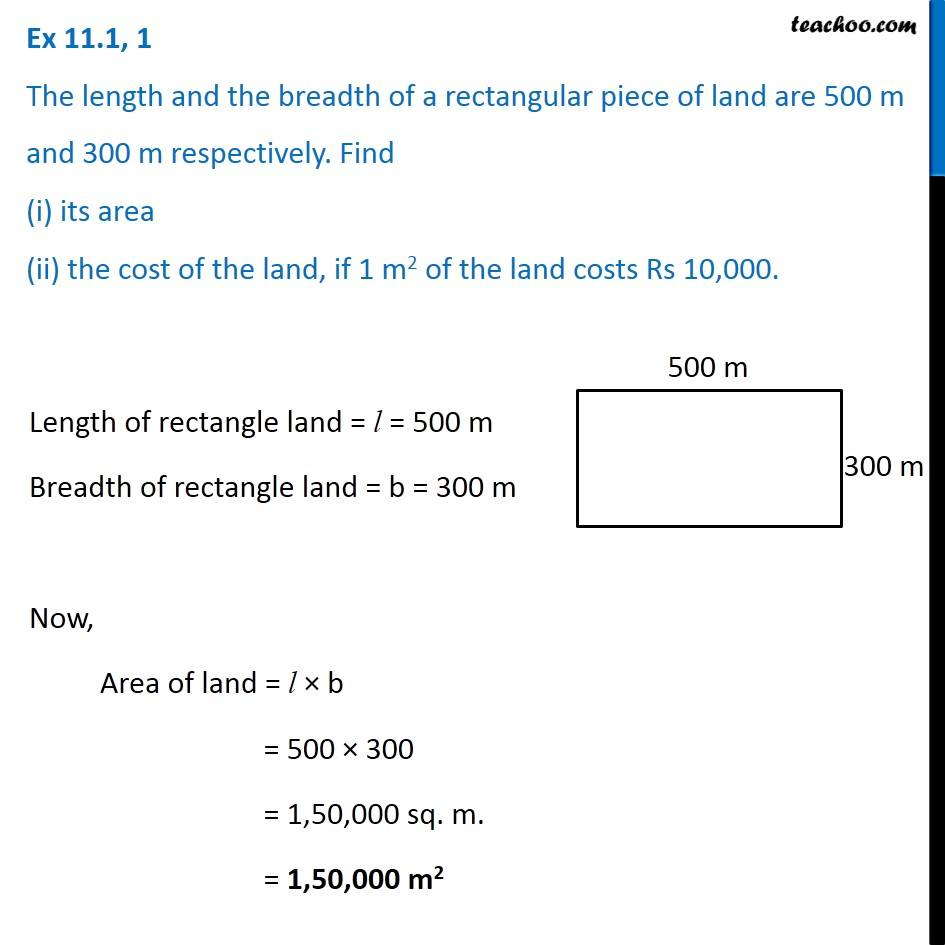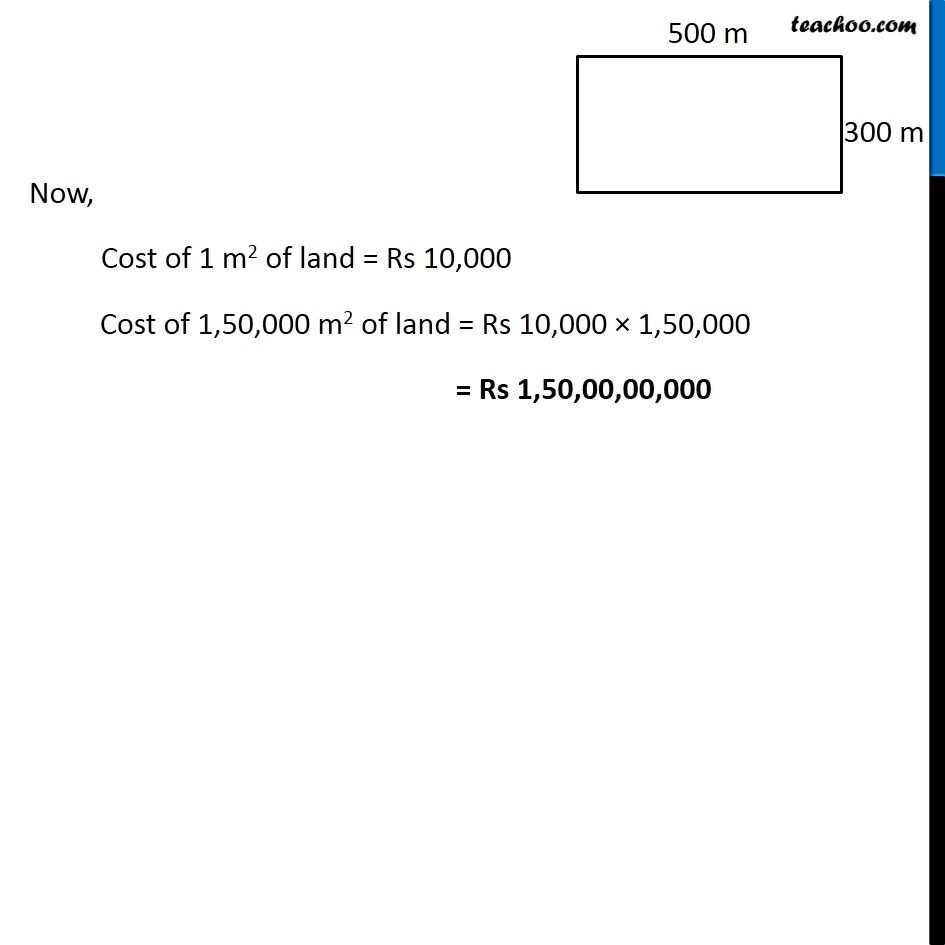Area of Square and Rectangles - Worksheet

Chapter 9 Class 7 Perimeter and Area
Serial order wiseLearn in your speed, with individual attention - Teachoo Maths 1-on-1 Class

### Transcript

Question 1 The length and the breadth of a rectangular piece of land are 500 m and 300 m respectively. Find (i) its area (ii) the cost of the land, if 1 m2 of the land costs Rs 10,000. Length of rectangle land = l = 500 m Breadth of rectangle land = b = 300 m Now, Area of land = l × b = 500 × 300 = 1,50,000 sq. m. = 1,50,000 m2 Now, Cost of 1 m2 of land = Rs 10,000 Cost of 1,50,000 m2 of land = Rs 10,000 × 1,50,000 = Rs 1,50,00,00,000